###### Descriptions and Examples for the POV-Ray Raytracerby Friedrich A. Lohmüller Elementary Geometry for Raytracing
Italiano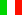Français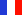Deutsch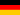Home
- POV-Ray Tutorial

- Geometrical Basics
for Raytracing

Right-angled Triangle
Pythagorean Theorem
Trigonometry Basics
Law of cosines
Equilateral Triangle
Regular Polygon
Polyhedron
Tetrahedron
Octahedron
Cube & Cuboid
Dodecahedron
Icosahedron
Cuboctahedron
Truncated Octahedron
Rhombicuboctahedron
Truncated Icosahedron
Circles
Tangent circles
Internal Tangents
External Tangents

- Geometric 3D Animations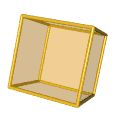## Cube and CuboidSome useful geometrical facts.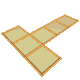In the following we write for the square root of a number the expression "sqrt(ZAHL)"
conforming to the syntax used in POV-Ray.

 Dimensions of a cube Length of an edge of the cube: a. The length of the diagonals of the faces:     d = sqrt (2) * a ; The length of the diagonals through a cube:     d = sqrt (3) * a ; The radius of circumsphere:     R = 1/2*sqrt( 3 ) * a ; Dimensions of a cuboid Length of the edges of the cubid: a, b, c. The length of a diagonal through a cuboid: d = sqrt ( a2 + b2 + c2 )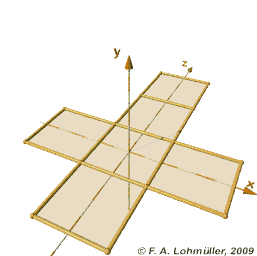Folding of a cube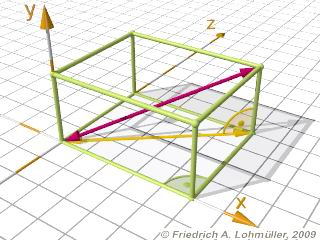Diagonals in a cuboid (rectangular parallelepiped)Folding of a cube

How to make this: The Folding of a Cube Net - 3D Animation with POV-Ray.
top

 © Friedrich A. Lohmüller, 2010 http://www.f-lohmueller.de